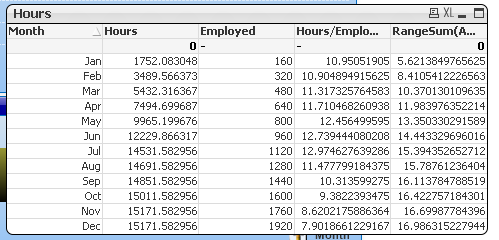# QlikView App Development

Discussion Board for collaboration related to QlikView App Development.

Highlighted
New Contributor III

## Calculating FTE dependent on Selections

Hello,

I'm having a hard time with calculating FTE (Full-time equivalents while including selections).

In calculating FTE, depending on dimension (time) you divide hours worked by 1920 (year), 160 (month), 480 (quarter), etc.

Now, I have a situation where it is possible to have more than 1 year, so therefore, I would say, 2015 and 2016 have been selected, sum hours from both years and then divide by (1920*2).

This makes things complicated when it comes to dynamic calculations.

For instance it is currently September 2016 so if I had that year and month selected I would calculate like so : sum(hours) / 9*160

If I had both 2015 and 2016 and September I would need the FTE of Jan - Sep for both years. Therefore, sum(hours) / (9*160)*2.

I think I have the logic down I'm just having trouble bringing it to life with expression(s). Any ideas?

Thanks,
Channing

11 RepliesMVP

## Re: Calculating FTE dependent on Selections

Sum(hours)/((Num(Month) * 160) * Count(DISTINCT Year))

New Contributor III

## Re: Calculating FTE dependent on Selections

Sunny,

This works great and is very close. Now, I need to figure out how to have a rolling sum of the hours worked from the previous month.

This is where  your expression has me now (I'd like to ignore those that fall under August as it's missing data and 160 is a default value):Thanks,

ChanningMVP

## Re: Calculating FTE dependent on Selections

Well would you be able to elaborate as to what your expected output is?

New Contributor III

## Re: Calculating FTE dependent on Selections

Yes,

I would like to add the hours from the previous month (creating a cumulative sum) just like that which is happening under the Employed field in my last image.

So if you refer to my image February's Hours would  be 1752.08+1737.48 and Employed would be 320 (as it is in the image). If this logic was in place I'd get the total of Jan - July hours to divide by 1120 Employed

I can probably use Set Analysis to omit anything beyond July (this data is outdated, but I'd be omitting any month that hasn't occurred yet).

Does this make more sense?MVP

## Re: Calculating FTE dependent on Selections

Try this:

RangeSum(Above(Sum(hours)/((Num(Month) * 160) * Count(DISTINCT Year)), 0, RowNo()))

Valued Contributor III

## Re: Calculating FTE dependent on Selections

Try using =Rangesum("4th column",rowno())New Contributor III

## Re: Calculating FTE dependent on Selections

The rangesum works! Now I can omit the late rmonths with Set Analysis. Is it possible to retain these range sum values upon the selection of let's say May? So, when I select May I get 9965.19Thanks,

ChanningMVP

## Re: Calculating FTE dependent on Selections

May be like this

RangeSum(Above(Sum({1}hours)/((Num(Only({1}Month)) * 160) * Count({1}DISTINCT Year)), 0, RowNo())) * Avg(1)

New Contributor III

## Re: Calculating FTE dependent on Selections

The numbers don't quite line up. I'm sort of at a loss.